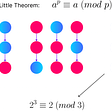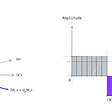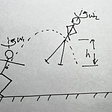# What is a number system really? Intro to the world of rings and fields.

There are a few types of numbers that we use every day (for more information https://katyhristova.medium.com/different-types-of-numbers-and-the-birth-of-i-22a994ee5fbd ).

## But.. what are the unifying properties of those? What makes a collection of numbers a legitimate number system?

Let’s think of the integers. Remember these are the positive whole numbers — 1,2,3, etc, as well as their negative counterparts — -1,-2,-3, etc and 0. The main purpose of a number system is to enable us to assign numerical values to various objects so we can:

(a) understand their structure

and

(b) distinguish between the different types of classes of objects.

For example, assigning lengths to the sides of a triangle can help us understand its perimeter and area, but also this enable us to classify it as equilateral, isosceles or scalene.

Except for being a quantitative measurement, what other properties do numbers have? What makes a collection of symbols a valid entity that we refer to as a number system? We will answer all these questions abstractly, but before that let us take a tour through the known sets of numbers and see what they’ve got in common and what makes them different.

The most basic operations we learn at school are — addition, subtraction, multiplication and division. When we take two whole numbers, let’s say 3 and 5, we want to be able to add them together, subtract one from the other, multiply and divide them and get a number as a result. If we do these basic operations, we see that 3+5=8, 5–3=2, 3–5=-2, 3×5=15 and 3÷5=0.6.

Remember we started with integers. If we look at the results, three out of the four operations give us an integer as a result too. If we move on to our next largest set of numbers — the rationals (integers plus fractions), and use the fact that all integers are also rational numbers, we can make some new observations. Firstly, all our “inputs” into the addition, subtraction, multiplication and division operations are integers and by extension — rationals. Secondly, all the four numbers we got as a result, i.e., our “outputs”, are also rationals. In other words, mathematically speaking, the set of rational numbers is closed under addition, subtraction, multiplication and division. The same applies for the sets of real and complex numbers.

Notice that all operations above (addition, subtraction, multiplication and division) have two inputs and one output. An operation of this form is called a binary operation. Hence, all the basic arithmetic operations are examples of the abstract notion of a binary operation.

## What other properties do numbers have?

We can group the four basic operations above into two groups. Put addition and subtraction together in one group and multiplication and division into another. The reason for doing this is that addition and subtraction are related in the same way as multiplication and division. More precisely, subtraction is inverse to addition and division is inverse to multiplication. Let me explain in more detail what this means.

Let’s go back to our example of inputing 3 and 5 into the binary operations. If we add 5 to 3, we get 8. Now if we take away 5 from 8 we get 3 — the number we started with. The same is true for multiplication and division — 3×5=15, then 15÷3=5. Also 5×3=15, 15÷5=3.

The inverse operation undos what the original operation has done. Thinking about how we paired the four operations, what we can deduce is that we only have two main operations — addition and multiplication, and the other two — subtraction and division, are their inverses.

Look at the examples:

3+5=8, 5+3=8, 3×5=15 and 5×3=15.

We see that 3+5=5+3 and 3×5=5×3. What this tells us is that when we perform those two operations, once we have chosen the numbers we want to input (3 and 5 in this case), the order of input does not matter. More precisely, swapping the place of the numbers we are adding or multiplying would make no difference to the result, it will not change our output.

In mathematical terms this property is called commutativity. We just established (by means of examples, not really by a mathematical proof) that the operations multiplication and addition are commutative for the integers. The same is true for the other main sets of numbers — rationals, reals and even the complex numbers.

So far we have been working with two numbers as inputs. But when we do addition or multiplication, we are not limited to only adding or multiplying two numbers. We can take more — three, four, five, ten, or in general any finite number of inputs.

Let us focus on having three inputs for now. Suppose I choose the numbers 3, 5 and 7 and I want to add them together. I can do this in two ways: I first add 3 and 5, then add 7 to the result, or I add 5 and 7 and then add 3 to the result. The order of performing the operation is mathematically encoded by using brackets. Whatever is inside the brackets has priority. So writing the above as an equation is:

(3+5)+7=8+7=15 and 3+(5+7)=3+12=15.

We can see that in both cases we get the same result. The same will happen if instead of addition we do multiplication. So the order of which pair we choose to first input into the binary operation doesn’t change the output. Mathematically this property is called associativity (of the binary operation). Associativity is important because it tells us what rules apply when we operate with triples, and by extension, with finitely many numbers.

Another special property that numbers have is called distributivity. This is a very useful rule which tells us how the two main operations — multiplication and addition, interact with one another. Distributivity is used when we either have brackets that we want to get rid of, or when we want to group parts of an algebraic expression together and create brackets. The first is what we call “opening brackets” or “expanding”, and the second is “simplification”.

Formally distributivity says that if we have three numbers, a,b, and c, the following holds:

(a+b)×c = a×c + b×c (“expand the brackets”).

Since the equal sign is a symmetric operator, the above of course implies that

a×c + b×c = (a+b)×c “simplify the algebraic expression”.

Let me show you an example (with numbers).

(3+5)×7= 3×7 + 5×7.

It may seem a silly thing to do, however, if we are solving equations (algebraic expressions with both numbers and letters), this property is very useful.

## What did we learn?

Let’s recap. All sets of numbers — integers, rational, real and complex, are closed under the binary operations addition and multiplication. The inverse of addition is called subtraction and the inverse of multiplication is called division. The integers are closed under subtraction too but not under division. The rational, real and complex numbers are closed under subtraction. Multiplication and addition are commutative operations, that obey the laws of associativity and distributivity.

All abstract mathematical structures with the above properties are called commutative rings. The fundamental idea is that those are structures closed under two binary operations, which are commutative, one (that we think of as addition) has inverses, they both are associative and distributive. If both binary operations have inverses, the commutative ring is what is called a field. The integers are a commutative ring but not a field, but the rational, real and complex numbers are fields.

If the operations are not commutative, the structure is called a non-commutative ring, or a ring. An example of a non-commutative ring are the quaternions. Remember that this is a 4-dimensional number system, obtained by adding 3 new abstract numbers i,j,k that satisfy a list of relations. Each quaternion can be represented as a+bi+cj+dk, where a,b,c,d are real numbers. The reason why it fails for the quaternions are the 9(of which we only list 6 here) fundamental relations which tell us that multiplying first I then j is not the same as first j then k. Written mathematically, the relations tell us ij=k, ji=-k, jk=i, kj=-i, ki=j and ik=-j.

We can see from those that if we calculate i times j, we get k. However, if we calculate, j times i, we get -k. And since -k is not the same as k, the results are different.

--

--

--

## More from Katerina Hristova

Writer | Copyeditor | Maths PhD

Love podcasts or audiobooks? Learn on the go with our new app.

## Squid Game Player Survival Probability in the Glass Bridge Game## Running with the Fibonacci Numbers## An Intuitive Introduction to Monte Carlo Simulations## The Colour of Numbers## Functionality of Miller-Rabin Primality Test## Understanding Grover’s Algorithm## Katerina Hristova

Writer | Copyeditor | Maths PhD

## Why Do I Keep Getting Static Shocks?## Why Is the Quad So Difficult in Figure Skating?## How close are the rationals and irrationals and what is the “most irrational number”?